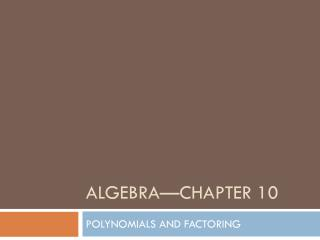DownloadDownload PresentationAlgebra—Chapter 10

# Algebra—Chapter 10

Télécharger la présentation## Algebra—Chapter 10

- - - - - - - - - - - - - - - - - - - - - - - - - - - E N D - - - - - - - - - - - - - - - - - - - - - - - - - - -
##### Presentation Transcript

1. Algebra—Chapter 10 POLYNOMIALS AND FACTORING

2. Section 10.1—Adding and subtracting polynomials

3. Standard form of a polynomial: • Terms are placed in descending order—largest degree to smallest • Example: 2x3 + 5x2 – 4x + 7 • Vocab: • Degree of each term: exponent on variable • Degree of polynomial: largest degree of its terms • Leading coefficient: number in front when in standard form

4. Degree of a polynomial • Find the degree of the following polynomials • x – 23 • 3x2 + 2x – 1 • x3 + 3x2 + 2x – 1 • x12 + 3x43 + 32

5. Naming polynomials • Can be named by terms • Monomial—one term • Binomial—two terms • Trinomial—three terms • Polynomial—more than 3 terms • Can be named by degree • Constant—degree 0 • Linear—Degree 1 • Quadratic—Degree 2 • Cubic—Degree 3 • Quartic—Degree 4

6. Adding polynomials • (5x3 – x + 2x2 + 7) + (3x2 + 7 – 4x) • (2x4 – 4x3 – 15x2 + 21x + 4) + (4x4 + 2x3 +17)

7. Try on your own… • (6x2 – x + 3) + (-2x + x2 – 7)

8. Subtracting Polynomials: • (2x3 + 5x2 – x + 8) – (x3 + 3x – 4) • (x2 – 8) – (7x + 4x2)

9. Try on your own: • (4x2 – 1) – (3x – 2x2)

10. Writing in standard form: • Write the expression in standard form: • 5x + 6 – 3x3 – 4x2

11. Guided practice p 579 7-12

12. Assignment • Section 10.1 • Page 580-581 • 14-38 evens • 65-66

13. Multiplying Polynomials—10.2

14. Review! • Try the following:

15. What if we are multiplying by more than 1 thing? • How does this work with two terms? • Visually?

16. Using Algebra Tiles… • Try to figure out the following: • (x+6)(x+2) • (x+1)(x+3) • (x+2)(x+2) • (x+3)(x+1)

17. Using Algebra Tiles… • Try to figure out the following: • (2x+1)(x+5) • (x+2)(2x+3) • (2x+1)(2x+1)

18. Is there an easier way to do this? • FOIL Method • A way to distribute binomials • In other words, multiply every term in the first group by every term in the second

19. Using FOIL… • Try to figure out the following: • (x+6)(x+2) • (x+1)(x+3) • (x+2)(x+2) • (x+3)(x+1)

20. Using FOIL… • Try to figure out the following: • (2x+1)(x+5) • (x+2)(2x+3) • (2x+1)(2x+1)

21. Does this work with larger groups? • Again…Foil is just a way to make sure we multiply all of the terms together

22. p.587#18-35

23. p.587#36-46, 52-53 • Due @ End of the hour! 

24. Special Products of Polynomials—10.3

25. What about FOIL? • FOIL is just an idea • Yes, it works for everything but is there an easier way? • In some cases, yes! • Recognition! • Example: 11 x 5 = ? • Did you show your work? No? Shortcut??

26. Special Product Patterns • Sum and Difference Pattern • Square of a Binomial

27. Special Product Patterns • (x-2)(x+2)

28. Special Product Patterns • (2x+1)(2x-1)

29. Special Product Patterns • (-3t+2)(-3t+2)

30. Special Product Patterns • (x+4)(x+4)

31. Special Product Patterns • (2x-3)(2x-3)

32. Special Product Patterns • (7a+2)(7a+2)

33. Special Product Patterns

34. Special Product Patterns

35. Guided Practice • Problems 6-14

36. p.593#16-38 Even

37. Solving Polynomials in Factored Form—10.4

38. Graph!

39. Solving Quadratics • Graphing… • Raise your hand if you LOVE graphing!  • Ok….so….not everyone’s favorite • Also, is it the most accurate? • Hmmm…..what if we had an easier way?!

40. Factored Form • If we take the equationand factor it, we end up with: • We will talk about where this comes from soon!  • What values of x make this true?

41. Zero Product Property • The product of two factors is zero iff at least one of the factors is zero • Technically…Let a and b be real numbers. If ab = 0, then z = 0 or b = 0

42. Steps for finding zeros • Set Equal to Zero • Factor! • These 2 will be done for you today… • Take each of the factors and set them equal to zero • Treat as separate equations • Write your solutions! • Smile! 

43. Examples

44. Examples

45. Examples

46. Examples

47. Examples

48. Examples

49. Guided Practice! • Problems 12-17